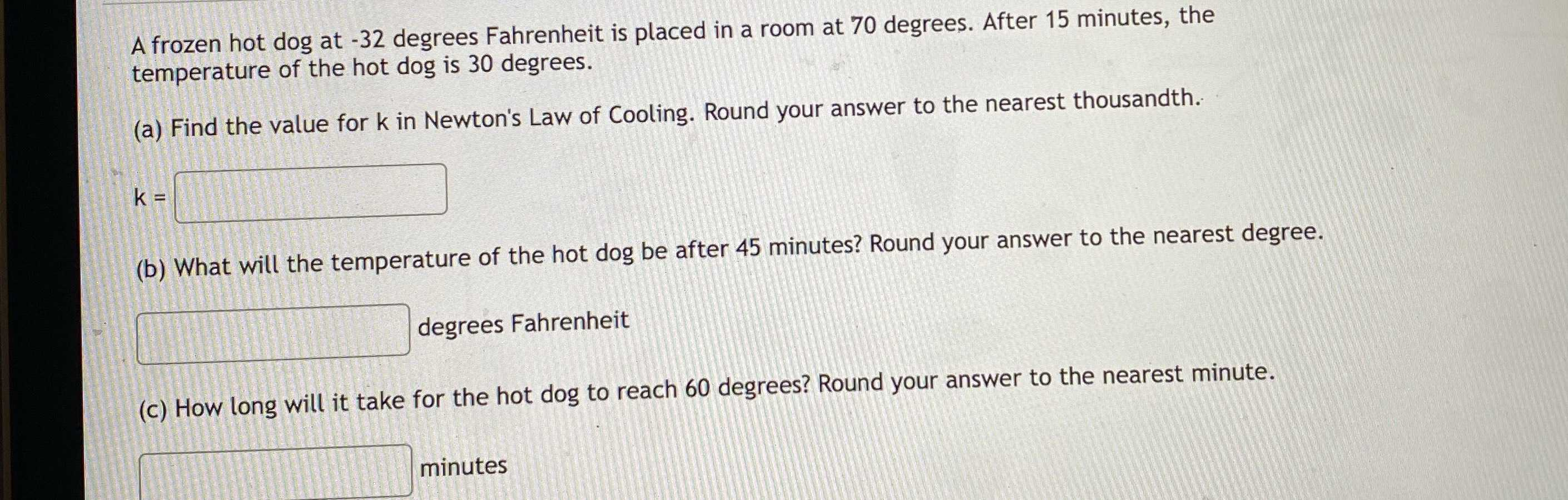### ¿Todavía tienes preguntas de matemáticas?

Pregunte a nuestros tutores expertos
Algebra
PreguntaA frozen hot dog at - $$32$$ degrees Fahrenheit is placed in a room at $$70$$ degrees. After $$15$$ minutes, the temperature of the hot dog is $$30$$ degrees.

(a) Find the value for $$k$$ in Newton's Law of Cooling. Round your answer to the nearest thousandth.

(b) What will the temperature of the hot dog be after $$45$$ minutes? Round your answer to the nearest degree.

(c) How long will it take for the hot dog to reach $$60$$ degrees? Round your answer to the nearest minute.